# Class 12 Maths NCERT Solutions for Chapter 5 Continuity and Differentiability Exercise 5.7### Continuity and Differentiability Exercise 5.7 Solutions

1. Find the second order derivatives of the function.
x2 + 3x + 2

Solution

Let  y = x2 + 3x + 2
Then,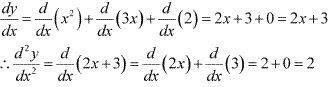2. Find the second order derivative of the function x20  .

Solution

Let, y = x20
Then,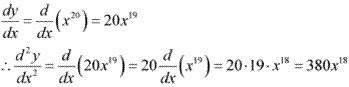3. Find  the second order derivatives of the function.
x. cos x

Solution

Let y = x . cos x
Then,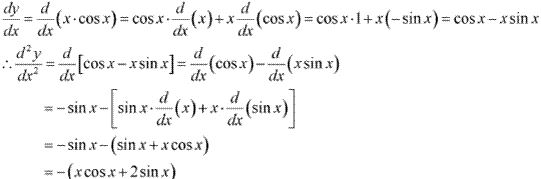4.  Find the second order derivatives of the function.
log x

Solution

Let y = log x
Then,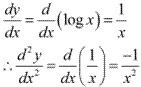5. Find the second order derivatives of the function.
x3 log x

Solution

Let y = x3 log x
Then,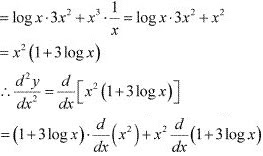= (1 + 3 log x) . 2x + x2 . 3/x
= 2x + 6x log x + 3x
= 5x + 6x log x
= x(5 + 6 log x)

6. Find the second order derivatives of the function.
ex sin 5x

Solution

Let y = ex sin 5x
Then,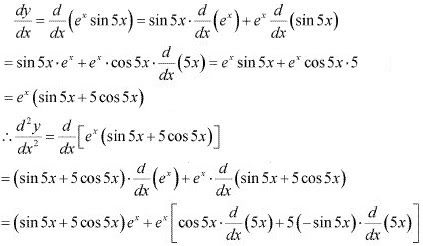= ex (sin 5x + 5 cos 5x) + ex (5cos 5x - 25 sin 5x)
= ex (10 cos 5x - 24 sin 5x) = 2ex (5 cos 5x - 12 sin 5x)

7. Find the second order derivatives of the function.
e6x cos 3x

Solution

Let y = e6x cos 3x
Then,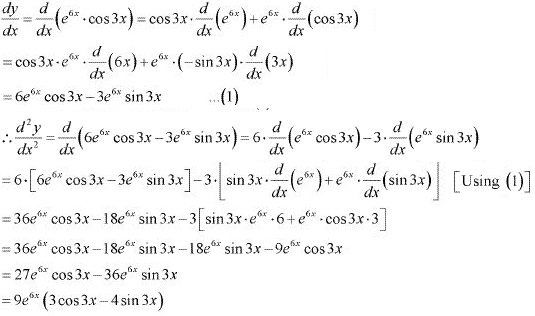8. Find the second order derivatives of the function.
tan-1 x

Solution

Let y = tan-1 x
Then,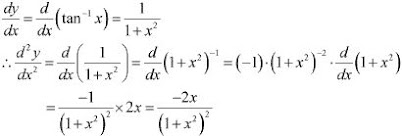9. Find the second order derivatives of the function.
log (log x)

Solution

Let y = log (log x)
Then,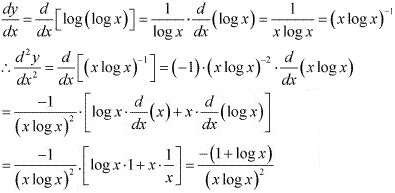10. Find the second order derivatives of the function.
sin (log x)

Solution

Let y = sin(log x)
The,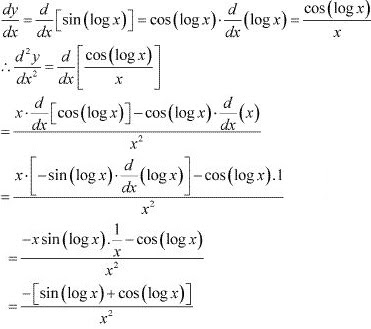11. If y = 5 cos x - 3 sin x, prove that (d2 y/dx2 ) + y = 0    .

Solution

It is given that, y = 5 cos x - 3 sin x
Then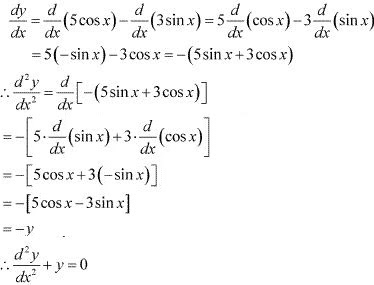∴ (d2 y/dx2 ) + y = 0
Hence, proved

12. If y = cos-1 x, Find d2 y/dx2  in terms of y alone.
Solution
It is given that, y = cos-1 x,
Then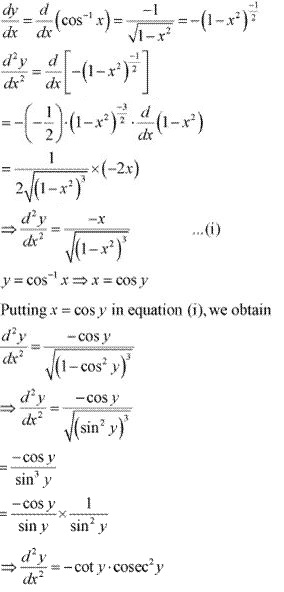13. If y = 3 cos (log x) + 4 sin (log x), show that x2 y2 + xy1 + y = 0
Solution
It is given that , y = 3 cos (log x) + 4 sin (log x),
Then,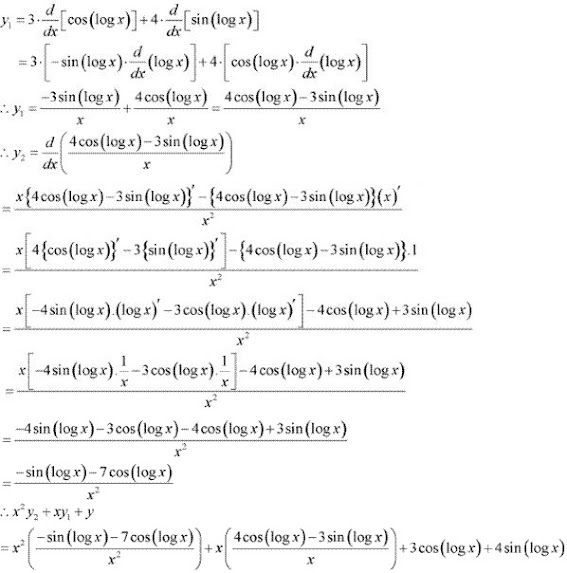14. If y = Aemx + Benx , show that d2 y/dx2 - (m + n) dy/dx + mny = 0
Solution
It is given that, y = Aemx + Benx
Then,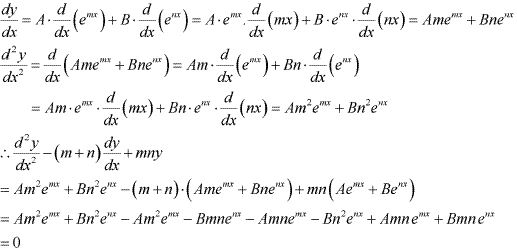Hence, proved.

15. If y = 500e7x + 600e-7x  , show that d2 y/dx2 = 49y
Solution
It is given that , y = = 500e7x + 600e-7x
Then,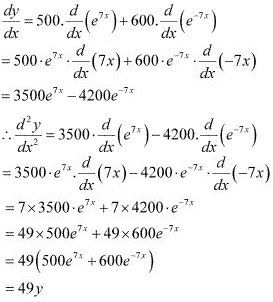16. If ey (x + 1) = 1, show that d2 y/dx2  = (dy/dx)2
Solution
The given relationship is ey (x + 1) = 1
ey (x + 1) = 1
⇒ ey = 1/(x + 1)
Taking logarithm on both the sides, we obtain
y = log . 1/(x + 1)
Differentiating this relationship with respect to x, we obtain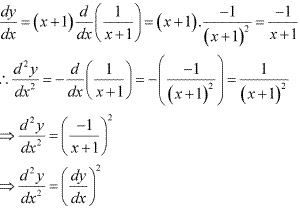Hence, proved.

17. If y = (tan–1 x)2  , show that (x2 + 1)2 y2 + 2x (x2 + 1) y1 = 2
Solution
The given relationship is y = (tan–1 x)2
Then,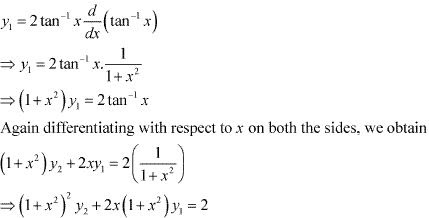Hence, proved.